## SHM p.13

### Visualizing the Wavefunctions

We have found the following wavefunction for our 3-d oscillator:with energy E'=4nr+2l+3.

What do these wavefunctions look like?

If l=0 the wavefunctions are spherically symmetric.

If l>0, the probability densities for these wavefunctions (but not the wavefunctions themselves) are independent of("cylindrically symmetric" for a cylinder whose axis is aligned with the z axis) so we only need to concern ourselves with their dependence onand r (i.e., for example the x-z plane). (The exp(im) dependence in the wavefunction cancels out in*.) The probability density for +m and -m are identical (since Yl,-m=(-1)mY*l,m ); the difference is only which way the particle is going around the z axis. Note that except for l=0 the wavefunction goes to zero as you approach the origin; the larger the value of l the fastergoes to zero. Note that unless m=0 the wavefunction is zero on the z axis; again larger |m| means a more disallowed z axis. For the largest possible |m| (i.e., ml) the probability is largely near the x-y plane. Finally, there are always nr radial nodes (zeros) [not counting the origin].

Below we plot the probability density for nr=2, l=2 ("d" wave) for m=0,1,2. For several of these plots the probability density is also plotted along a particular line.

### m=0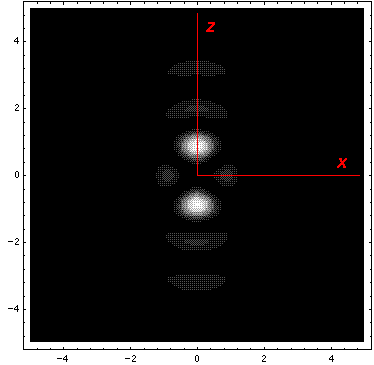### m=1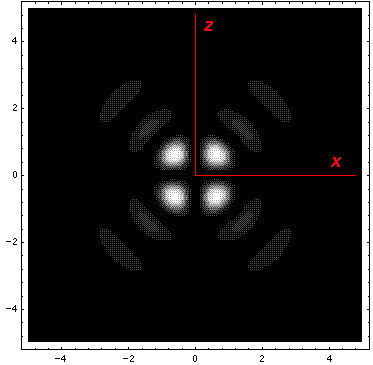### m=2Note that the probability is mostly confined to the z=0 plane (i.e., the x-y plane) Here is what the probability density looks like in that plane; like all of these solutions it issymmetric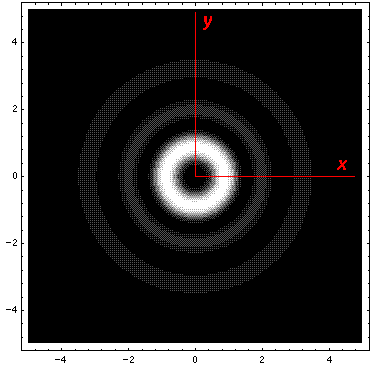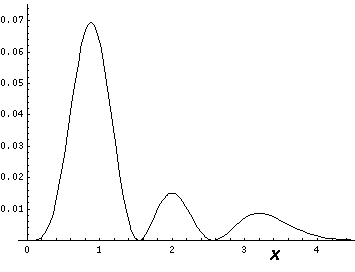All the the above solutions have E'=15. Here is a collection of solutions with E'=27.

### nr=2,l=8,m=0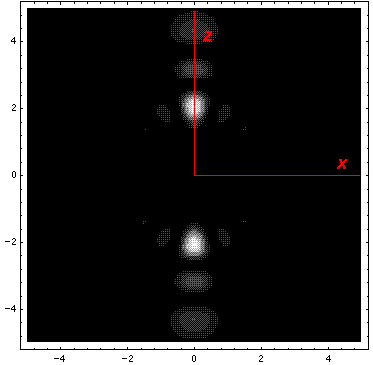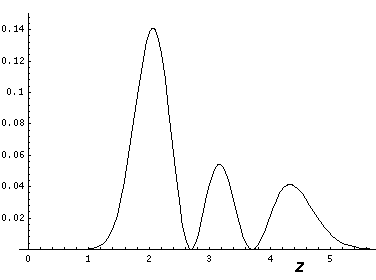### nr=2,l=8,m=8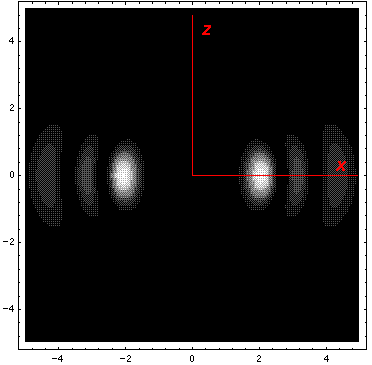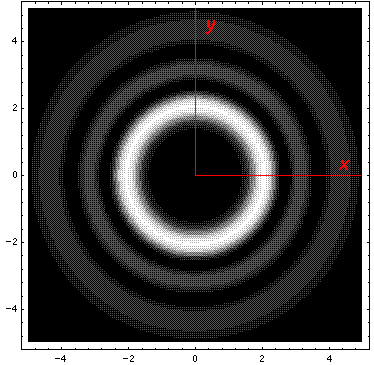### nr=6,l=0,m=0

This l=0 solution is spherically symmetric, so all planes going through the origin have the same probability density. For this particular case there is such a large difference between the probability density at the origin and for the last few oscillations near the edge of the classically allowed region, that I have had to "stretch" the scales. In the case of the density plot, contours are spaced equally in logarithm (here a factor of 2 separates adjacent contours) rather than arithmetically (constant differences) spaced; in the case of the plot of probability density vs r, I've included a "50×" magnified plot of the large r probability density.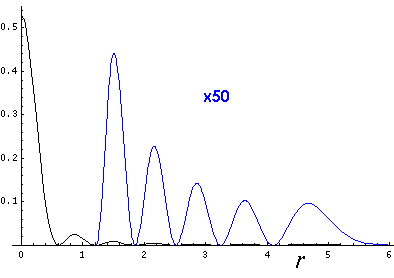Because the angular dependence is "simple" we can usefully plot the wavefunction just as a function of r. Here are "stacked wavefunction" plots for l=0,1,3:The red line is the classical "effective potential"= l(l+1)/r2+r2 which includes the "centrifugal barrier" l(l+1)/r2 for a particle with l total angular momentum (essentially) l. Because of the centrifugal barrier, non-zero l wavefunctions are excluded from the origin.